# VBA COUNT

This tutorial will show you how to use the Excel COUNT function in VBA

The VBA COUNT function is used to count the number of cells in your Worksheet that have values in them. It is accessed using the WorksheetFunction method in VBA.

## COUNT WorksheetFunction

The WorksheetFunction object can be used to call most of the Excel functions that are available within the Insert Function dialog box in Excel. The COUNT function is one of them.

``````Sub TestCountFunctino
Range("D33") = Application.WorksheetFunction.Count(Range("D1:D32"))
End Sub``````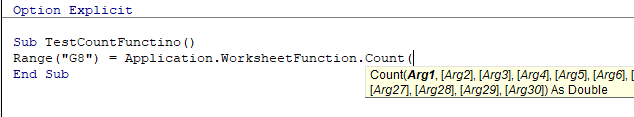You are able to have up to 30 arguments in the COUNT function. Each of the arguments must refer to a range of cells.

This example below will count how many cells are populated with values are in cells D1 to D9

``````Sub TestCount()
Range("D10") = Application.WorksheetFunction.Count(Range("D1:D9"))
End Sub``````

The example below will count how many values are in a range in column D and in a range in column F. If you do not type the Application object, it will be assumed.

``````Sub TestCountMultiple()
Range("G8") = WorksheetFunction.Count(Range("G2:G7"), Range("H2:H7"))
End Sub``````

## Assigning a Count result to a Variable

You may want to use the result of your formula elsewhere in code rather than writing it directly back to and Excel Range.  If this is the case, you can assign the result to a variable to use later in your code.

``````Sub AssignCount()
Dim result As Integer
'Assign the variable
result = WorksheetFunction.Count(Range("H2:H11"))
'Show the result
MsgBox "The number of cells populated with values is " & result
End Sub``````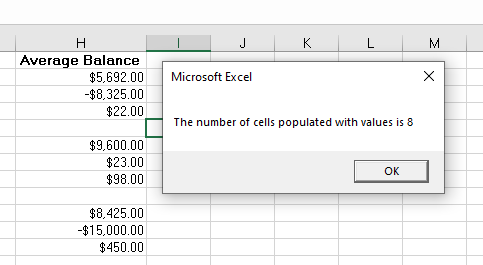## COUNT with a Range Object

You can assign a group of cells to the Range object, and then use that Range object with the WorksheetFunction object.

``````Sub TestCountRange()
Dim rng As Range
'assign the range of cells
Set rng = Range("G2:G7")
'use the range in the  formula
Range("G8") = WorksheetFunction.Count(rng)
'release the range object
Set rng = Nothing
End Sub``````

## COUNT Multiple Range Objects

Similarly, you can count how many cells are populated with values in multiple Range Objects.

``````Sub TestCountMultipleRanges()
Dim rngA As Range
Dim rngB as Range
'assign the range of cells
Set rngA = Range("D2:D10")
Set rngB = Range("E2:E10")
'use the range in the formula
Range("E11") = WorksheetFunction.Count(rngA, rngB)
'release the range object
Set rngA = Nothing
Set rngB = Nothing
End Sub``````

## Using COUNTA

The count will only count the VALUES in cells, it will not count the cell if the cell has text in it. To count the cells which are populated with any sort of data, we would need to use the COUNTA function.

``````Sub TestCountA()
Range("B8) = Application.WorksheetFunction.CountA(Range("B1:B6"))
End Sub``````

In the example below, the COUNT function would return a zero as there are no values in column B, while it would return a 4 for column C. The COUNTA function however, would count the cells with Text in them and would return a value of 5 in column B while still returning a value of 4 in column C.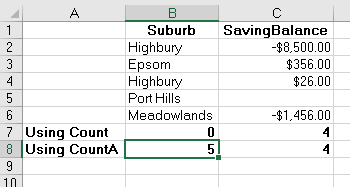## Using COUNTBLANKS

The COUNTBLANKS function will only count the Blank Cells in the Range of cells – ie cells that have no data in them at all.

``````Sub TestCountBlank()
Range("B8) = Application.WorksheetFunction.CountBlanks(Range("B1:B6"))
End Sub``````

In the example below, column B has no blank cells while column C has one blank cell.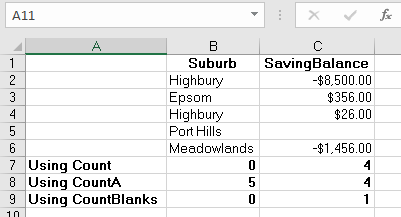Stop searching for VBA code online. Learn more about AutoMacro - A VBA Code Builder that allows beginners to code procedures from scratch with minimal coding knowledge and with many time-saving features for all users!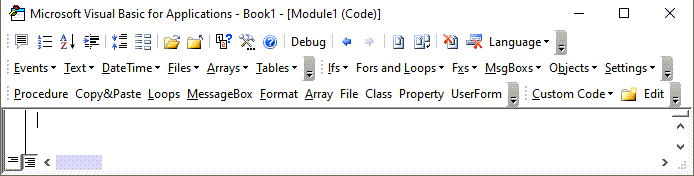## Using the COUNTIF Function

Another worksheet function that can be used is the COUNTIF function.

``````Sub TestCountIf()
Range("H14") = WorksheetFunction.CountIf(Range("H2:H10"), ">0")
Range("H15") = WorksheetFunction.CountIf(Range("H2:H10"), ">100")
Range("H16") = WorksheetFunction.CountIf(Range("H2:H10"), ">1000")
Range("H17") = WorksheetFunction.CountIf(Range("H2:H10"), ">10000")
End Sub``````

The procedure above will only count the cells with values in them if the criteria is matched – greater than 0, greater than 100, greater than 1000 and greater than 10000.  You have to put the criteria within quotation marks for the formula to work correctly.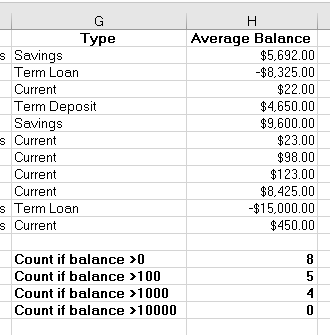When you use the WorksheetFunction to count the values in a range in your worksheet, a static value is returned, not a flexible formula. This means that when your figures in Excel change, the value that has been returned by the WorksheetFunction will not change.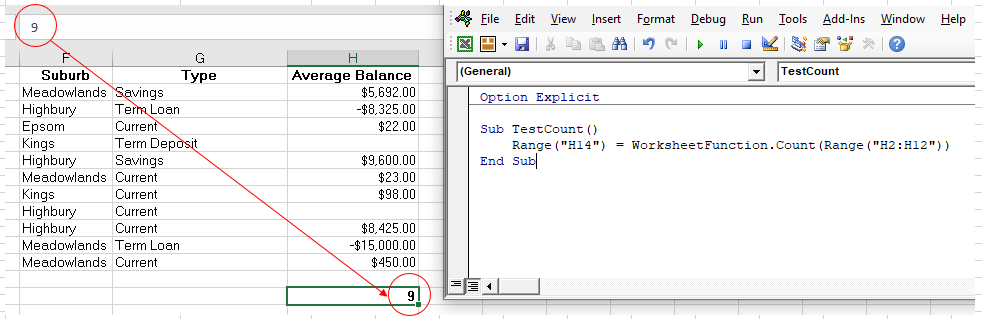In the example above, the procedure TestCount has counted up the cells in column H where a value is present.  As you can see in the formula bar, this result is a figure and not a formula.

If any of the values change therefore in the Range(H2:H12), the results in H14 will NOT change.

Instead of using the WorksheetFunction.Count, you can use VBA to apply a Count Function to a cell using the Formula or FormulaR1C1 methods.

### Using the Formula Method

The formula method allows you to point specifically to a range of cells eg: H2:H12 as shown below.

``````Sub TestCountFormula
Range("H14").Formula = "=Count(H2:H12)"
End Sub``````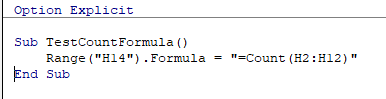VBA Programming | Code Generator does work for you!

### Using the FormulaR1C1 Method

The FromulaR1C1 method is more flexible in that it does not restrict you to a set range of cells. The example below will give us the same answer as the one above.

``````Sub TestCountFormula()
Range("H14").Formula = "=Count(R[-9]C:R[-1]C)"
End Sub``````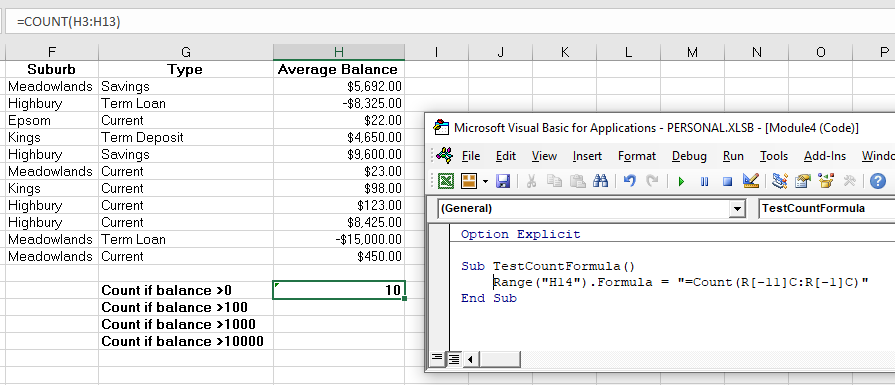However, to make the formula more flexible, we could amend the code to look like this:

``````Sub TestCountFormula()
ActiveCell.FormulaR1C1 = "=Count(R[-11]C:R[-1]C)"
End Sub``````

Wherever you are in your worksheet, the formula will then count the values in the 12 cells directly above it and place the answer into your ActiveCell. The Range inside the COUNT function has to be referred to using the Row (R) and Column (C) syntax.

Both these methods enable you to use Dynamic Excel formulas within VBA.

There will now be a formula in H14 instead of a value.